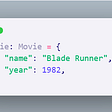# Solution 1: Some important Flux tips and Flux utilities to make writing Flux easier

`from(bucket:"items")    |> range(start:-1h)      |> aggregateWindow(every: 1m, fn: mean())`
`multByX = (x) => (column, tables=<-) =>  tables  |> mean(column:column)     |> map(fn: (r) => ({ r with _value: r._value * x})) from(bucket: "my-bucket")   |> range(start: v.timeRangeStart, stop: v.timeRangeStop)   |> filter(fn: (r) => r["_measurement"] == "cpu")   |> filter(fn: (r) => r["_field"] == "usage_system")   |> limit(n: 10)   |> aggregateWindow(every: v.windowPeriod, fn: multByX(x:2.0))`

# Solution 2: Learning about memory optimizations and new pushdown patterns to optimize your Flux scripts

• `|> count()`
• `|> sum()`
• `|> first()`
• `|> last()`
• `|> min()`
• `|> max()`
• `|> mean()`
• `|>group() |> count()`
• `|>group() |> sum()`
• …etc.
• `|>window() |> count()`
• `|>window() |> sum()`
• …etc.
• `|>aggregateWindow(fn: count)`
• `|>aggregateWindow(fn: sum)`
• …etc.

# Solution 4: Task writing recommendations.

`option task = {name: “rateTask”, every: 1h}rate = from(bucket: “items”)  |> range(start: -task.every)  |> filter(fn: (r)  => (r._measurement == “totalItems”))  |> filter(fn: (r)  => (r._field == “items”))  |> group(columns: [“itemGroupName”])  |> aggregateWindow(fn: sum, every: task.every)  |> map(fn: (r) => {    return: _time: r._time,  _stop: r._stop, _start: r._start, _measurement: task.name, newTag: “rate”, _value: r._value, itemGroupName: itemGroupName,   }})rate   |> to(bucket: “newItems”)Copy`
`from(bucket: “neItems”)  |> range(start: -task.every)  |> filter(fn: (r)  => (r._measurement == “rateTask”)  |> filter(fn: (r)  => (r.newTag == “rate”)`
`option task = {name: "Downsampling CPU", every: 1m}data = from(bucket: "my-bucket")|> range(start: -task.every)|> filter(fn: (r) => r._measurement == "my_measurement")data|> mean()|> set(key: "agg_type",value: "mean_temp")|> to(bucket: "downsampled", org: "my-org", tagColumns: ["agg_type"])data|> count()|> set(key: "agg_type",value: “count_temp")|> to(bucket: "downsampled", org: "my-org", tagColumns: ["agg_type"])`

# Next steps for tacking hurdles for intermediate Flux users

--

--

--

## More from Anais Dotis

Love podcasts or audiobooks? Learn on the go with our new app.

## Why I choose freeCodeCamp!!## Slow Agile — Part Two: the Psychology of Numbers## Linux Basic Commands## Finding The Most Important Sentences Using TF-IDF by Python## Playing With KMS Key Policies## Saving gas with Propane tools## How Orbitron Works## “Static” and runtime type checking for python dict at same time## How Docker eased up my life?